# Preparing Physical Structures for Electromagnetic Simulation

Jump to: navigation, search

## Assigning Material Properties to the Physical Structure

In CubeCAD, geometric objects are simply grouped together by their color. They do not have any physical properties. However, in all of EM.Cube's computational modules, you need to assign physical properties to each geometric object. In these modules, geometric objects are grouped together by their common physical properties as well as their color. The types of physical properties may differ in different computational modules, but they are typically related to the material properties or boundary conditions.

From an electromagnetic modeling point of view, materials are categorized by the constitutive relations or boundary conditions that relate electric and magnetic fields. In general, an isotropic material medium is macroscopically characterized by four constitutive parameters:

• Permittivity (ε) having units of F/m
• Permeability (μ) having units of H/m
• Electric conductivity (σ) having units of S/m
• Magnetic conductivity (σm) having units of Ω/m

The permittivity and permeability of a material are typically related to the permittivity and permeability of the free space as follows:

$\epsilon = \epsilon_r \epsilon_0$
$\mu = \mu_r \mu_0, \quad \quad$

where ε0 = 8.85410×10-12 F/m are fundamental constants, μr = 1.257×10-6 H/m, and εr and μr are called relative permittivity and permeability of the material, respectively.

The constitutive parameters relate the field quantities in the material medium:

$\mathbf{D} = \epsilon \mathbf{E}, \quad \quad \mathbf{J} = \sigma \mathbf{E}$
$\mathbf{B} = \epsilon \mathbf{H}, \quad \quad \mathbf{M} = \sigma_m \mathbf{H}$

where E and H are the electric and magnetic fields, respectively, D is the electric flux density, also known as the electric displacement vector, B is the magnetic flux density, also known as the magnetic induction vector, and J and M are the electric and magnetic current densities, respectively.

The electric conductivity and magnetic conductivity parameters represent the material losses. In frequency-domain simulations under a time-harmonic (ejωt) field assumption, it is often convenient to define a complex relative permittivity and a complex relative permeability in the following manner:

$\epsilon_r = \epsilon^{\prime}_r -j\epsilon^{\prime\prime}_r = \epsilon^{\prime}_r -j\frac{\sigma}{\omega \epsilon_0} = \epsilon^{\prime}_r (1 - j \tan \delta )$
$\mu_r = \mu^{\prime}_r -j\mu^{\prime\prime}_r = \mu^{\prime}_r - j\frac{\sigma_m}{\omega \mu_0} = \mu^{\prime}_r (1 - j \tan \delta_m)$

where ω = 2πf, and f is the operational frequency, and the electric and magnetic loss tangents are defined as follows:

$\tan \delta = \epsilon^{\prime\prime}_r / \epsilon^{\prime}_r$
$\tan \delta_m = \mu^{\prime\prime}_r / \mu^{\prime}_r$

Three special media are frequently encountered in electromagnetic problems:

Medium εr μr σ σm
Free Space 1.0 1.0 0.0 0.0
Perfect Electric Conductor (PEC) 1.0 1.0 0.0
Perfect Magnetic Conductor (PMC) 1.0 1.0 0.0

## Using EM.Cube's Materials List

EM.Cube provides a preloaded database of popular and widely used materials.

Click the Materials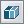button of the Simulate Toolbar or select Menu → Simulate → Materials... or use the keyboard shortcut Ctrl+T to open EM.Cube's Materials List dialog. You can view the various material entries and their constitutive parameter values. You can also type in the first letter of a material to find it. For example, typing V selects Vacuum in the list.

In many of EM.Cube's modules you have to define material properties for as part of definition of object groups. In the property dialog of some of these groups you will see a Material button. If you click this button, it will open up EM.Cube's Material List. Select any material from the list and close the dialog by clicking its OK. The selected material's properties will then fill the corresponding parameter fields automatically.

## Defining New Custom Materials

The contents of EM.Cube's Materials List and the listed material parameters are based on published and cataloged data as well as some manufacturer data sheets. They are not definitive by any means. In fact, certain materials like different types of glass, wood, plastic, etc. may have a range of material parameters depending on the manufacturer, temperature, water content and lots of other factors. EM.Cube allows you to define new materials and add them to the Materials List. You can also modify the parameters of a standard or custom material.

To add a new material, follow these steps:

• Click the New... button of the Materials dialog open the "Add New Material" dialog.
• Choose a name and enter values for the four constitutive parameters of the new material.
• Check the check box labeled Specify Loss Tangent if you want to define a loss tangent instead of an electric conductivity value. In that case, the value for σ is calculated based on the center frequency of your project and reflected in the greyed-out sigma box.
• Click the OK button of the dialog to return to the Materials dialog. You will see the name of your new name in the Materials List sorted alphabetically. The new material entry is display in blue fonts.

To edit a custom material, select it from the list and click the Edit button of Materials dialog. To modify the properties of a preloaded standard material, select its name from the list and click the arrow on the New... button of the Materials dialog and select the Modify Existing Material option from the dropdown list.A new custom material entry in the Materials List.

## Defining an Excitation Source

An electromagnetic boundary value problem requires an excitation source that typically appears as the right-hand-side (RHS) term of a differential or integral equation. A source can be an impressed electric or magnetic field or an impressed electric or magnetic current that produces an impressed electric or magnetic field. Sources can be lumped, confined to a single point or an infinitesimal region, or they can be distributed over a sizable area or volume of the computational domain.

EM.Cube provides a large variety of source types for the excitation of your physical structure. Some source type have identical definitions in different computational modules. For example, plane waves behave exactly the same way in EM.Tempo, EM.Illumina, EM.Libera or EM.Picasso. Or Hertzian short dipole sources are identical in EM.Terrano, EM.Illumina, EM.Libera or EM.Picasso. On the other hand, some source types apply only to certain computational modules. For example, Gaussian beam sources appear only in EM.Tempo. In all of EM.Cube's computational modules except for EM.Ferma, the source types are listed under the Sources section of the navigation tree. In these modules, the sources either exist on their own as virtual objects or they are associated with the existing geometric objects in the project workspace. In EM.Ferma, electrostatic and magnetostatic sources like volume charges or wire currents are defined as geometric objects side by side of material objects in the project workspace.

In each of EM.Cube's computational modules, you need to choose the right source type depending on the purpose of your simulation and based on the observables you define for your project. For example, for computing the radar cross section (RCS) of a target, you need a plane wave source. If you are interested in computing the S/Z/Y parameters of your structure, then you have to choose a source type like a gap or lumped source that supports a "Port Definition" observable. For a detailed description of each source type in the context of its application, please refer to the manual of individual computational modules.

## Exciting Multiport Structures in EM.Cube

In many electromagnetic modeling problems, your physical structure can be treated as a one-port, two-port or multiport circuit. Each port may contain a voltage or current source with an internal resistance or may have been terminated in a resistive or complex impedance. To characterize such circuits, a number of parameters are typically defined. The most widely used port characteristics are scattering (S) parameters, impedance (Z) parameters and admittance (Y) parameters. One-port circuits are typically excited using a voltage source in series with an internal resistance. The voltage source might be lumped and confined to a single point or to an infinitesimal region, or it can be distributed on a specified surface. The EM.Cube modules that offer voltage sources are EM.Tempo, EM.Picasso and EM.Libera. In other words, there three modules are the only ones that allow you to compute S/Z/Y parameters of a physical structure. Each modules offers a number of special source types for this purpose.

Soure Type Module Host Types Primary Port Parameters
Lumped Source EM.Tempo PEC and thin wire lines S-parameters
Distributed Source EM.Tempo Virtual rectangular region (rectangle strip) S-parameters
Microstrip Port Source EM.Tempo PEC rectangle strips S-parameters
CPW Port Source EM.Tempo PEC rectangle strips S-parameters
Coaxial Port Source EM.Tempo PEC cylinders S-parameters
Waveguide Port Source EM.Tempo Hollow box objects S-parameters
Strip Gap Circuit EM.Picasso Rectangular metal (PEC or conductive sheet) traces Y-parameters
Strip Gap Circuit EM.Picasso Rectangular slot traces Z-parameters
Probe Gap Circuit EM.Picasso Embedded PEC vias Y-parameters
Strip Gap Circuit EM.Libera - Surface MoM PEC strips Y-parameters
Wire Gap Circuit EM.Libera - Wire MoM PEC and thin wire lines and polylines Y-parameters

Each of the above source types is used to compute a certain type of port characteristics, i.e. either S parameters, or Y parameters or Z parameters. Once one set of parameters are computed, the other parameters are calculated based on well-known matrix relationships.

If your physical structure has two or more sources, but you have not defined any ports, all the lumped sources excite the structure simultaneously. However, when you assign N ports to the sources, then you have a multiport structure that is characterized by an N×N scattering matrix, an N×N impedance matrix, and an N×N admittance matrix. To calculate these matrices, EM.Cube uses a binary excitation scheme in conjunction with the principle of linear superposition. In this binary scheme, the structure is analyzed N times. Each time one of the N port-assigned sources is excited, and all the other port-assigned sources are turned off. The N solution vectors that are generated through the N binary excitation analyses are finally superposed to produce the actual solution to the problem. However, in this process, EM.Cube also calculates all the port characteristics.

For example, EM.Tempo primarily computes the S-parameters, which relates the incident and reflected power waves at the port of the structure as follows:

$\mathbf{ [b] = [S][a] }$

where the incident and reflected power waves ai and bi at port i are related to the voltage Vi across port i and the current Ii flowing into port i in the following manner:

$a_i = \frac{V_i + Z_i I_i}{2\sqrt{|Re(Z_i)|}}, \quad\quad b_i = \frac{V_i - Z_i^* I_i}{2\sqrt{|Re(Z_i)|}}$

and Zi is the reference or characteristic impedance of port i. For the computation of the S-parameters in EM.Tempo, the source associated with each port is excited separately with all the other ports turned off. Due to the existence of internal source resistances, tuning the other sources off is indeed equivalent to terminating the other ports in their characteristics impedances (matched ports). When the jth port is excited, all the Sij parameters are calculated together based on the following definition:

$S_{ij} = \sqrt{\frac{|Re(Z_i)|}{|Re(Z_j)|} } \cdot \frac{V_j - Z_j^*I_j}{V_i+Z_i I_i}$

The sweep loop then moves to the next port until all ports have been excited. The other parameters are then calculated as:

$\mathbf{ [Z] = [\sqrt{Z_0}] \cdot ([U]+[S]) \cdot ([U]-[S])^{-1} \cdot [\sqrt{Z_0}] }$
$\mathbf{ [Y] = [Z]^{-1} }$

where $\mathbf{[U]}$ is the identity matrix of order N, and [$\mathbf{\sqrt{Z_0}}$] is a diagonal matrix whose diagonal elements are the square roots of port characteristic impedances.

In EM.Picasso and EM.Libera, the Y-parameters are computed directly from the gap voltage sources. A gap source on a metal strip or a metal wire behaves like a series voltage source with a prescribed strength (of 1V and zero phase by default) that creates a localized discontinuity on the path of electric current flow (Js). At the end of the MoM simulation, the electric current passing through the voltage source (Vs) is computed and integrated to find the total input current (Iin). From this one can calculate the input admittance as

$Y_{in} = \frac{I_{in}}{V_s} = \frac {\int_W \hat{y} \cdot \mathbf{J_s} \, dy} {V_s}$

for gap sources on metal traces, where the line integration is performed across the width of the metal strip. In the case of a wire gap, the current solution is indeed the total current.

It is important to note that the input admittance defined at a gap source port is referenced to the two terminals of the voltage source connected across the gap as shown in the figure below. This is different than the input admittance that one may normally define for a microstrip port, which is referenced to the substrate's ground. To resolve this problem, you can place a gap source on a metal strip line by a distance of a quarter guide wavelength (λg/4) away from its open end. Note that λg = 2π/β, where β is the propagation constant of the metallic transmission line. As show in the figure below, the impedance looking into an open quarter-wave line segment is zero, which effectively shorts the gap source to the planar structure's ground. The gap admittance in this case is identical to the input admittance of the planar structure.Definition of different input impedances at the gap location.Placing a gap source a quarter guide wavelength away from the open end of a feed line to effectively short it to the ground at the gap location.

In EM.Picasso, a probe source on a PEC via behaves like a series voltage source with a prescribed strength (of 1V and zero phase by default) that creates a localized discontinuity on the path of the vertical electric current flow (Jp). At the end of the planar MoM simulation, the electric current passing through the voltage source is computed and integrated to find the total input current. From this one can calculate the input admittance as

$Y_{in} = \frac{I_{in}}{V_s} = \frac {\int_S \hat{z} \cdot \mathbf{J_p} \, ds} {V_s}$

where the surface integration is performed over the cross section of the via. The case of a probe source placed on a PEC via that is connected to a ground plane is more straightforward. In this case, the probe source's gap discontinuity is placed at the middle plane of the PEC via. If the via is short, it is meshed using a single prismatic element, which is connected to the ground from one side and to the metal strip line from the other. Therefore, the probe admittance is equal to that of the structure at a reference plane that passed through the host via.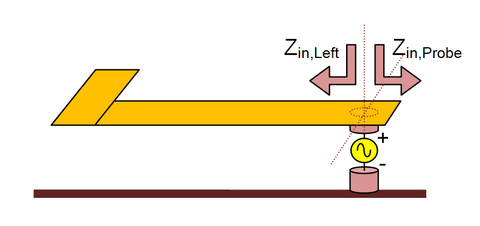Input impedance of a probe source on a PEC via connected to a ground plane.

In the case of gap sources on metal traces and probe sources on PEC vias, turning a source off means shorting a series voltage source. The electric currents passing through these sources are then found at each port location, and the admittance parameters are found as follows:

$I_m = \sum_{n=1}^N Y_{mn} V_n, \quad \quad Y_{mn} = \frac{I_m}{V_n} \bigg|_{V_k=0, k \ne n}$

The other parameters are then calculated as:

$\mathbf{ [S] = [Y_0] \cdot ([Z]-[Z_0]) \cdot ([Z]+[Z_0])^{-1} \cdot [Z_0] }$
$\mathbf{ [Z] = [Y]^{-1} }$

where [Z0] is a diagonal matrix whose diagonal elements are the port characteristic impedances, and [Y0] is a diagonal matrix whose diagonal elements are the port characteristic admittances.

On the other hand, a gap source on a slot trace behaves like a shunt current source with a prescribed strength (of 1A and zero phase by default) that creates a localized discontinuity on the path of magnetic current flow (Ms). At the end of a planar MoM simulation, the magnetic current passing through the current source (Is) is computed and integrated to find the total input voltage across the current filament (Vin). From this one can calculate the input impedance as

$Z_{in} = \frac{V_{in}}{I_s} = \frac{\int_W \hat{y} \cdot \mathbf{M_s} \,dy} {V_s} = \frac{\int_W E_y \, dy}{V_s}$

The same principle applies to the gap sources on slot traces. The figure below shows how to place two gap sources with opposite polarities a quarter guide wavelength away from their shorted ends to calculate the correct input impedance of the CPW line looking to the left of the gap sources. Note that in this case, you deal with shunt filament current sources across the two slot lines and that the slot line carry magnetic currents. The end of the slot lines look open to the magnetic currents, but in reality they short the electric field. The quarter-wave CPW line acts as an open circuit to the current sources.

In the case of gap sources on slot traces, turning a source off means opening a shunt filament current source. The magnetic currents passing through the source locations, and thus the voltages across them, are then found at all ports, and the impedance parameters are found as follows:

$V_m = \sum_{n=1}^N Z_{mn} I_n, \quad \quad Z_{mn} = \frac{V_m}{I_n} \bigg|_{I_k=0, k \ne n}$Placing two oppositely polarized gap sources a quarter guide wavelength away from the short end of a CPW line to effectively create an open circuit beyond the gap location.

## Calculating Scattering Parameters Using Prony's Method

EM.Picasso provides a special source type called Scattering Wave Port that is specifically intended for computing the S-parameters of planar structures. This is done by analyzing the current distribution patterns on the port transmission lines. The discontinuity at the end of a port line (junction region) gives rise to a standing wave pattern in the line's current distribution. From the location of the current minima and maxima and their relative levels, one can determine the reflection coefficient at the discontinuity, i.e. the S11 parameter. A more rigorous technique is Prony’s method, which is used for exponential approximation of functions. A complex function f(x) can be expanded as a sum of complex exponentials in the following form:

$f(x) \approx \sum_{n=1}^N c_i e^{-j\gamma_i x}$

where ci are complex coefficients and γi are, in general, complex exponents. From the physics of transmission lines, we know that lossless lines may support one or more propagating modes with pure real propagation constants (real γi exponents). Moreover, line discontinuities generate evanescent modes with pure imaginary propagation constants (imaginary γi exponents) that decay along the line as you move away from the location of such discontinuities.

In practical planar structures, each port line normally supports one, and only one, dominant propagating mode. Multi-mode transmission lines are seldom used for practical RF and microwave applications. Nonetheless, each port line carries a superposition of incident and reflected dominant-mode propagating signals. An incident signal, by convention, is one that propagates along the line towards the discontinuity, where the phase reference plane is usually established. A reflected signal is one that propagates away from the port plane. Prony's method is used by EM.Picasso to extract the incident and reflected propagating and evanescent exponential waves from the standing wave data. From the knowledge of the amplitudes (expansion coefficients) of the incident and reflected dominant propagating modes at all ports, the scattering matrix of the multi-port structure is then calculated. In Prony's method, the quality of the S-parameter extraction results depends on the quality of the current samples and whether the port lines exhibit a dominant single-mode behavior. Clean current samples can be drawn in a region far from sources or discontinuities, typically a quarter wavelength away from the two ends of a feed line.Minimum and maximum current locations of the standing wave pattern on a microstrip line feeding a patch antenna.

## Modeling Coupled Sources & Ports

Sources can be coupled to each other to model coupled strip lines (CPS) on metal traces or coplanar waveguides (CPW) on slot traces. Similarly, probe sources may be coupled to each other. Coupling two or more sources does not change the way they excite a planar structure. It is intended only for the purpose of S parameter calculation. The feed lines or vias which host the coupled sources are usually parallel and aligned with one another and they are all grouped together as a single transmission line represented by a single port. This single "coupled" port then interacts with other coupled or uncoupled ports.

You couple two or more sources using the Port Definition Dialog. To do so, you need to change the default port assignments. First, delete all the ports that are to be coupled from the Port List of the dialog. Then, define a new port by clicking the Edit button of the dialog. This opens up the Add Port dialog, which consists of two tables: Available sources on the left and Associated sources on the right. A right arrow --> button and a left arrow <-- button let you move the sources freely between these two tables. You will see in the "Available" table a list of all the sources that you deleted earlier. You may even see more available sources. Select all the sources that you want to couple and move them to the "Associated" table on the right. You can make multiple selections using the keyboard's Shift and Ctrl keys. Closing the Add Port dialog returns you to the Port Definition dialog, where you will now see the names of all the coupled sources next to the name of the newly added port.When not using EM.Cube's wizards, it is the user's responsibility to set up coupled ports and coupled transmission lines properly. For example, to excite the desirable odd mode of a coplanar waveguide (CPW), you need to create two rectangular slots parallel to and aligned with each other and place two gap sources on them with the same offsets and opposite polarities. To excite the even mode of the CPW, you use the same polarity for the two collocated gap sources. Whether you define a coupled port for the CPW or not, the right definition of sources will excite the proper mode. The couple ports are needed only for correct calculation of the port characteristics.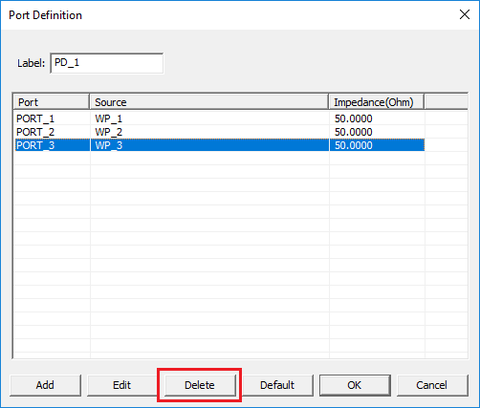Deleting port GS_3 that is to be coupled to port GS_2.Reassigning sources to ports and defining coupled ports in EM.Picasso.EM.Picasso's Port Definition dialog showing an independent port and two coupled ports.

## Modeling Finite-Sized Source Arrays

You can define arrays of sources on arrays of legitimate host objects. If your project in EM.Tempo has a line array object, you can define a lumped source array associated with it. If your project in EM.Picasso has an array of rectangle strips or an array of PEC vertical via objects, you can define and place a gap array or an array of probe sources on them, respectively. Similarly, if your project in EM.Libera has a line array object, a polyline array object, or an array of long, narrow rectangle strips, you can define and place a line gap array or a strip gap array on them, respectively. In all of these cases, the legitimate array objects will also be listed as an eligible object for source placement. A lumped or gap source will then be placed on each element of the array. All the individual sources will have identical direction and offset.

You can prescribe certain amplitude and/or phase distribution over the array of gap or probe sources. By default, all the gap or probe sources have identical amplitudes of 1V (or 1A for the slot case) and zero phase. The available amplitude distributions to choose from include Uniform, Binomial and Chebyshev and Date File. In the Chebyshev case, you need to set a value for minimum side lobe level (SLL) in dB. You can also define Phase Progression in degrees along all three principal axes. You can view the amplitude and phase of individual sources by right clicking on the top Sources item in the Navigation Tree and selecting Show Source Label from the contextual menu.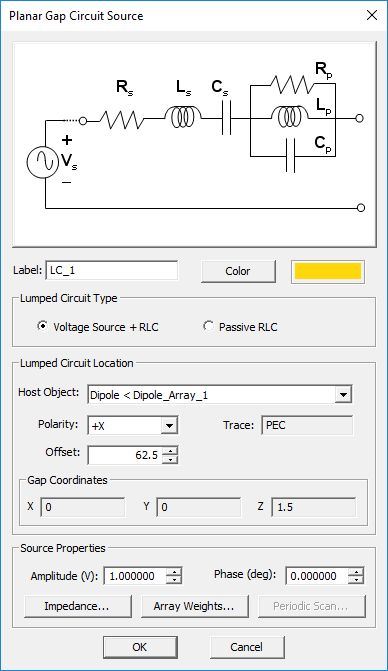Defining gap sources on an array of rectangle strip objects.Defining gap sources on an array of rectangle strip objects with a Chebyshev amplitude distribution.

In the data file option, the complex amplitude are directly read in from a data file using a real - imaginary format. When this option is selected, you can either improvise the complex array weights or import them from an existing file. In the former case click the New Data File button. This opens up the Windows Notepad with default formatted data file that has a list of all the array element indices with default 1+j0 amplitudes for all of them. You can replace the default complex values with new one and save the Notepad data file, which brings you back to the Gap Source dialog. To import the array weights, click the Open Data File button, which opens the standard Windows Open dialog. You can then select the right data file from the one of your folders. It is important to note that the data file must have the correct format to be read by EM.Cube. For this reason, it is recommended that you first create a new data file with the right format using Notepad as described earlier and then save it for later use.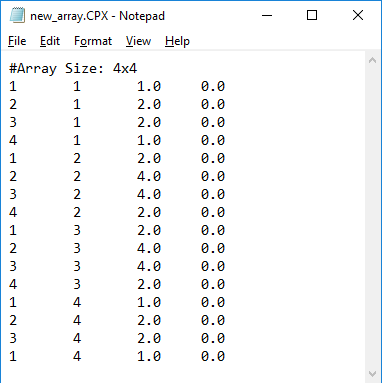Defining gap source array weights using a data file.

## Beam Steering in Periodic Phased Array Structures

In EM.Tempo, a periodic structure can be excited using various source types. Exciting the unit cell structure using a lumped source, a waveguide source, or a distributed source, you can model an infinite periodic antenna array. For most practical antenna types, you excite your periodic structure with a lumped source or waveguide source. In this case, you can define a port for the lumped source or waveguide source and calculate the S11 parameter or input impedance of the periodic antenna array. You can also compute the near-field and far-field data.

EM.Tempo's periodic FDTD simulator uses periodic boundary conditions (PBC) to model an infinite periodic array. All the periodic replicas of the unit cell structure are excited. In this case, you can impose a phase progression across the infinite array to steer its beam. You can do this from the property dialog of the lumped source or waveguide source. At the bottom of the Lumped Source Dialog or Waveguide Source Dialog, there is a section titled Periodic Beam Scan Angles. This section is grayed out when the project structure is not periodic. You can enter desired beam scan angle values for both Theta and Phi in degrees. To visualize the radiation pattern of the beam-steered array, you have to define a finite-sized array factor. You do this in the "Impose Array Factor" section of the Radiation Pattern Dialog.For large θ scan angles, the periodic FDTD time marching loop may take far more time steps to converge.

When a periodic planar structure is excited using a gap or probe source, it acts like an infinite periodic phased array. All the periodic replicas of the unit cell structure are excited. You can even impose a phase progression across the infinite array to steer its beam. You can do this from the property dialog of the gap or probe source. At the bottom of the Gap Source Dialog or Probe Source Dialog, there is a section titled Periodic Beam Scan Angles. You can enter desired values for Theta and Phi beam scan angles in degrees. To visualize the radiation patterns of a beam-steered antenna array, you have to define a finite-sized array factor in the Radiation Pattern dialog. You do this in the Impose Array Factor section of this dialog. The values of Element Spacing along the X and Y directions must be set equal to the value of Periodic Lattice Spacing along those directions.

## A Review of Linear & Nonlinear Passive & Active Devices

The Resistor Device

A resistor is a lumped device that dissipates power and converts electric energy to heat. A simple resistor is made of a bar of resistive material with a rectangular cross section and two metallic contacts at its two ends. A resistor is characterized by its resistance, which has units of Ohm (Ω) and is defined as

$R = \frac{V}{I} = \frac{- \int_C \mathbf{E(r)} . \mathbf{dl} }{ \int\int_S \sigma \mathbf{E(r)} . \mathbf{ds} }$

where V is the voltage across the resistor and I is the current passing through it. In the above equation, C represents an open curve (path), S represents an open surface like a plane, and E(r) is the electric field.

The resistance of a simple resistive bar with electric conductivity σ, length l and cross section area A is given by

$R = \frac{1}{\sigma} \frac{l}{A}$

The time-domain relationship between the voltage and current of a resistor obey Ohm's law:

$v(t) = R i(t)$

Under the assumption of time-harmonic excitation, the frequency-domain relationship can be expressed in phasor form:

$V = R I = Z_R I$

This means that the impedance of a resistor is pure real.

The Capacitor Device

A capacitor is a lumped device that stores electric energy. A simple capacitor is made of two parallel metal plates separated by a dielectric spacer. A capacitor is characterized by its capacitance, which has units of Farad (F) and is defined as

$C = \frac{Q}{V} = \frac{ \int\int_{S_o} \epsilon \mathbf{E(r)} . \mathbf{ds} }{ \int_C \mathbf{E(r)} . \mathbf{dl} }$

where Q is the total charge on the capacitor plates and V is the voltage across the two plates. In the above equation, C represents an open curve (path) and So represents a closed surface like a box.

The capacitance of a simple parallel plate capacitor with plate surface area A, plate spacing d and relative permittivity εr is given by

$C = \epsilon_r \epsilon_0 \frac{d}{A}$

The time-domain relationship between the voltage and current of a capacitor is given by:

$i(t) = C \frac{dv(t)}{dt}$

Under the assumption of time-harmonic excitation, the frequency-domain relationship can be expressed in phasor form:

$I = j\omega C V = Y_C V$

This means that the impedance of a capacitor is pure imaginary.

The Inductor Device

An inductor is a lumped device that stores magnetic energy. A simple inductor is made of a wire coil. An inductor is characterized by its inductance, which has units of Henry (H) and is defined as

$L = \frac{\Phi_H}{I} = \frac{ \int\int_S \mu \mathbf{H(r)} . \mathbf{ds} }{ \oint_{C_o} \mathbf{H(r)} . \mathbf{dl} }$

where ΦH is the total magnetic flux passing through the inductor and I is the current in the wire. In the above equation, Co represents a closed curve (loop), S represents an open surface like a plane, and H(r) is the magnetic field.

The inductance of a simple air-filled coil inductor with N turns, a circular cross section area A, and end-to-end length l is given by

$L = \mu_0 N^2 \frac{A}{l}$

The time-domain relationship between the voltage and current of an inductor is given by:

$v(t) = L \frac{di(t)}{dt}$

Under the assumption of time-harmonic excitation, the frequency-domain relationship can be expressed in phasor form:

$V = j\omega L I = Z_L I$

This means that the impedance of an inductor is pure imaginary.

The Nonlinear Diode Device

A diode is a rectifying device. The time-domain relationship between the voltage and current of a diode is given by the nonlinear equation:

$i(t) = I_s \left( e^{\frac{kv(t)}{nqT}} - 1 \right)$

where Is is called the saturation current, k = 1.3806488 × 10-23m2kg.s-2K-1 is the Boltzmann constant, q = 1.60217657 × 10-19 is the electron charge, T is the temperature in degrees Kelvin, and n is called the "Ideality Factor" and has a value between 1 and 2.

A diode is a directional device marked by positive anode and negative cathode terminals. It allows the flow of positive current in the direction from anode to cathode, but it blocks the flow of current in the opposite direction.

## Modeling Lumped Elements in the MoM Solvers

In both of EM.Cube's MoM-based computational modules, EM.Picasso and EM.Libera, the concept of lumped element has been generalized to an "Active Lumped Circuit", which consists of an ideal voltage source together with a series-parallel RLC combination as shown in the figure below. The combination is placed across a gap discontinuity located on a rectangle strip object or on a wire or on a vertical PEC via. You have the choice to set the source voltage to zero and make it a passive load. You can also selectively define the series and shunt RLC elements as you desire. For example, an active voltage source with a series resistor in EM.Picasso or EM.Libera would function like a lumped source in EM.Tempo, which has a built-in source resistance as part of its definition.A generalized active lumped circuit consisting of an ideal voltage source and a series-parallel RLC combination.

In both EM.Picasso and EM.Libera, a lumped element is treated as a complex impedance in the frequency domain. Therefore, the complex-valued Ohm's law is enforced as a boundary condition at the gap discontinuity that is associated with the location of the lumped element. If a lumped element is placed on a PEC line object or on a PEC rectangle strip object, it is treated as a series connection. The boundary condition at the location of the lumped element is:

$V_{gap} = Z_L I_{in} + V_s\quad\quad\quad\quad \int_{\delta} \hat{x}\cdot \mathbf{E_{gap}} \, dx = V_s + Z_L \int_W \hat{y} \cdot \mathbf{J_s} \, dy$

where ZL is the total impedance across the two terminals of the series element.Defining a series lumped element on a PEC strip line.

If the lumped element is placed on a slot trace, it is treated as a shunt connection that creates a current discontinuity. In this case, the magnetic current across the gap is continuous, and the boundary condition at the location of the lumped element is:

$I_{gap} = Y_L V_{in} + I_s \quad\quad\quad\quad \int_{\delta} J_Y^{filament} \, dx = I_s + Y_L \int_W E_y \, dy$

$\int_{\delta} \hat{x}\cdot\hat{n} \times (\mathbf{H_{gap}^+ - H_{gap}^-}) \, dx = I_s + Y_L \int_W \hat{y}\cdot\mathbf{M_s} \, dy$

where YL is the total admittance across the two terminals of the shunt element.

If a lumped element is placed on a PEC via that is connected to a metal strip from one side and to a PEC ground plane from the other end, it is indeed as a series connection across a gap discontinuity at the middle plane of the via. If the via is short, it is meshed using a single prismatic element. In that case, the lumped element in effect shunts the metal strip to the ground. The boundary condition at the location of the lumped element across the PEC via is:

$V_{gap} = Z_L I_{in} + V_s \quad\quad\quad\quad \int_{\delta} \hat{z}\cdot \mathbf{E_{gap}} \, dz = V_s + Z_L \int_S \hat{z} \cdot \mathbf{J_p} \, ds$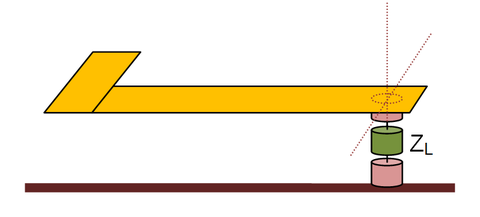Using a shunt lumped element on a PEC via to terminate a metallic strip line.

## EM.Cube Mesh Types

EM.Cube's computational modules use a number of different mesh generation schemes to discretize your physical structure. These include linear, surface and volume mesh generators.

Mesh Generator Mesh Type Supporting Module(s)
Adaptive Yee Mesh Volume Mesh EM.Tempo
Fixed-Cell Brick Mesh Volume Mesh EM.Tempo, EM.Ferma
Triangular Surface Mesh Surface Mesh EM.Illumina, EM.Libera, EM.Picasso, EM.Terrano
Hybrid Planar Mesh Surface Mesh EM.Picasso
Facet Mesh Surface Mesh EM.Terrano
Wireframe Mesh Linear Mesh EM.Libera

The objects of your physical structure are discretized based on a specified mesh density. The default mesh densities of EM.Tempo, EM.Picasso, EM.Libera and EM.Illumina are expressed as the number of cells per effective wavelength. Therefore, the resolution of the default mesh in these modules are frequency-dependent. You can also define the mesh resolution using a fixed cell size or fixed edge length specified in project units. The mesh density of EM.Terrano is always expressed in terms of cell edge length. The mesh resolution of EM.Ferma is always specified as the fixed cell size. All of EM.Cube's computational modules have default mesh settings that usually work well for most simulations.

The accuracy of the numerical solution of an electromagnet problem depends greatly on the quality and resolution of the generated mesh. As a rule of thumb, a mesh density of about 10-30 cells per effective wavelength usually yields satisfactory results. Yet, for structures with lots of fine geometrical details or for highly resonant structures, higher mesh densities may be required. Also, the particular simulation data that you seek in a project also influence your choice of mesh resolution. For example, far field characteristics like radiation patterns are less sensitive to the mesh density than the near-field distributions on a structure with a highly irregular shape and a rugged boundary.

## Working with EM.Cube's Mesh Generators

The mesh generation process in EM.Cube typically involves the following steps:

1. Setting the mesh properties.
2. Generating the mesh.
3. Verifying the integrity of the mesh.
4. Refining the mesh.

All the mesh generators of EM.Cube's individual computational modules have default settings, which produce a reasonably fine discretization of your physical structure. The most important mesh parameter is the mesh resolution. This can be expressed either as a global mesh density, i.e. the number of cells per wavelength, or by a cell size or maximum cell edge length. Many of EM.Cube mesh types can be customized at the local level of object groups or even at the level of individual objects.

To generating and viewing the mesh of your physical structure in any EM.Cube module, follow the steps below:

1. Click the Show Meshbutton of the Simulate Toolbar.
2. EM.Cube's "Mesh View" mode is enabled, and a mesh of your physical structure in the currently active EM.Cube module appears in the project workspace.
3. In the "Mesh View" mode, you can perform view operations like rotate view, pan or zoom, but you cannot create new objects or edit existing ones.
4. To exit the mesh view mode, press the keyboard's Esc key or click the Show Meshbutton once again.

Once a mesh is generated, it stays in the memory until the structure is changed or the mesh density or other settings are modified. Every time you view mesh, the one in the memory is displayed. You can force EM.Cube to create a new mesh from the ground up by selecting the menu item Simulate → Discretization → Regenerate Mesh or by right clicking on the Mesh item in the "Discretization" section of the navigation tree and selecting Regenerate from the contextual menu.

To customize the mesh of your physical structure or change some of the mesh settings in each EM.Cube module, follow the steps below:

1. Click the Mesh Settings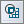button of the Simulate Toolbar.
2. The Mesh Settings dialog of the currently active EM.Cube module opens up.
3. From the Host drop-down list, select a line object. Note that only line parallel to one of the three principal axes are listed.
4. By default, the lumped source is placed at the midpoint of the host line object. You can modify the Offset parameter, which is measured from the start point of the line and is always positive.
5. Click the OK button of the dialog to return to the project workspace.

You can change the mesh algorithm from the dropdown list labeled Mesh Type if there is more than one option. You can also enter a different value for Mesh Density in cells per effective wavelength (λeff). For each value of mesh density, the dialog also shows the average "Cell Edge Length" in the free space.

## The Triangular Surface Mesh Generator

EM.Illumina, EM.Libera, EM.Terrano and EM.Picasso use a triangular surface mesh to discretize the structure of your project workspace. All these modules assume an unbounded, open-boundary computational domain, wherein the physical structure is placed against some sort of background medium with infinite extents. It is important to note that only finite-extent surfaces or the surface of finite-extent volumes are discretized. The surface mesh generating algorithm tries to produce regularized triangular cells with almost equal surface areas across the entire structure.Two ellipsoids of different compositions.Trinagular surface mesh of the two ellipsoids.

The following general rules apply to EM.Cube's surface mesh generators:

• The surface mesh generator merges all the objects that belong to the same group in the navigation Tree using the Boolean Union operation before meshing. The union operation is carried out temporarily and solely for the purpose of mesh generation.
• As a result of the Boolean union operation, all the overlapping objects are transformed into a single consolidated object leading to a contiguous and consistent mesh in the transition and junction areas between connected objects.
• In general, objects of the same CAD category can be "unioned". For example, surface objects can be merged together, and so can solid objects. However, a surface object and a solid in general do not merge.
• In general, objects that belong to different groups on the navigation tree are not merged during mesh generation even if they have the same material composition and physically overlap.
• Only EM.Libera's surface mesh generator creates a special junction mesh between overlapping objects that have different material compositions.
• All Polymesh objects as well as EM.Terrano's terrain objects are considered already discretized and are not re-meshed once again by the surface mesh generator.

## Controlling the Resolution & Quality of the Surface Mesh

You can control the average mesh cell size using the "Mesh Density" parameter. By default, the mesh density of EM.Illumina and EM.Libera is expressed in terms of the free-space wavelength. The default mesh density is 10 cells per wavelength. This usually creates slightly more than 100 regular triangular cells per squared wavelength. The default mesh density of EM.Picasso is expressed in terms of the effective wavelength, which takes into account the material properties of a planar structure's substrate layers. The default planar mesh density is 20 cells per effective wavelength. Alternatively, you can base the definition of the mesh density on "Cell Edge Length" expressed in project units. EM.Terrano's mesh density is always expressed in cell edge length and its default value is 100 units. This large edge length is intended to create the fewest number of triangular facets on cubic objects with rectangular faces.

You can change the value of Mesh Density from the Mesh Settings dialog and generate a triangular mesh with a higher or lower resolution.

Surface mesh generation in EM.Cube is a two-step process. First, a tessellated version of an object in your project workspace is created. Then, the tessellated object undergoes a surface re-meshing to generate regularized triangular cells. This process is fairly straightforward in the case of flat planar structures. For curved surfaces and curved solid objects, the quality of the initial tessellation of the object is very important and directly affects the quality of the final surface mesh. You can access some additional mesh parameters by clicking the Tessellation Options button of the Mesh Settings dialog. In the Tessellation Options dialog, you can change Curvature Angle Tolerance expressed in degrees, which as a default value of 15°. This parameter can affect the shape of the mesh especially in the case of solid CAD objects. It determines the apex angle of the triangular cells of the primary tessellation mesh which is generated initially before cell regularization. Lower values of the angle tolerance result in a less smooth and more pointed mesh of curved surface like a sphere.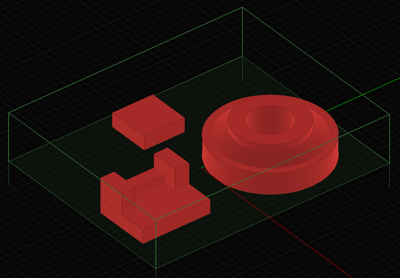Three building objects with different basic and composite shapes in EM.Terrano.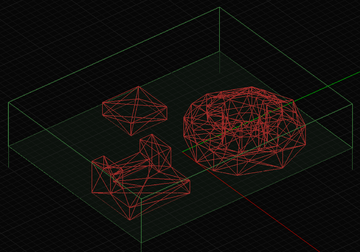The default mesh of the three building objects in EM.Terrano with an edge length of 100m and a curvature angle tolerance of 45°.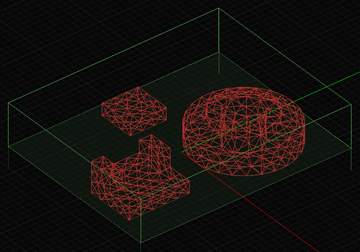The refined mesh of the three building objects in EM.Terrano with an edge length of 10m and a curvature angle tolerance of 10°.

## Locking the Mesh Density of Object Groups

The mesh density is a global parameter. It controls the resolution of the mesh of all the geometric objects in your project workspace. Some of EM.Cube's modules like EM.Tempo and EM.Picasso refine the mesh in material regions or on certain traces based on the material properties of those regions. This leads to a non-uniform mesh from region to region, e.g., from a free-space region to a material block.

Another way of local mesh control is to lock the mesh density of certain object groups. In that case, the locked mesh density takes precedence over the global mesh density. Locking the mesh can be useful for isolated object groups that may require a higher (or lower) mesh resolution. You can lock the local mesh density by accessing the property dialog of any object group or material group from the navigation tree. Check the box labeled Fix Max Mesh Density and enter a value for the mesh density different than the global mesh density of your project.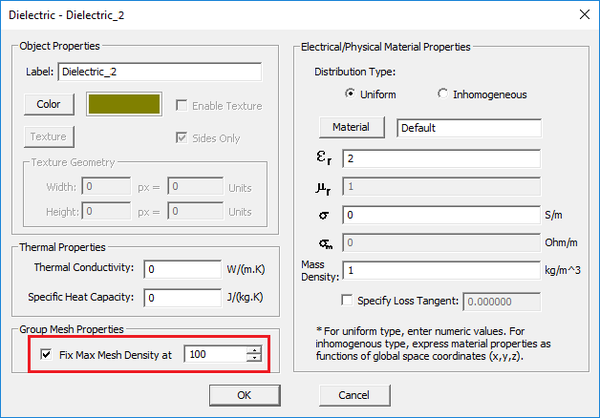Locking the mesh density in the property dialog of a material group.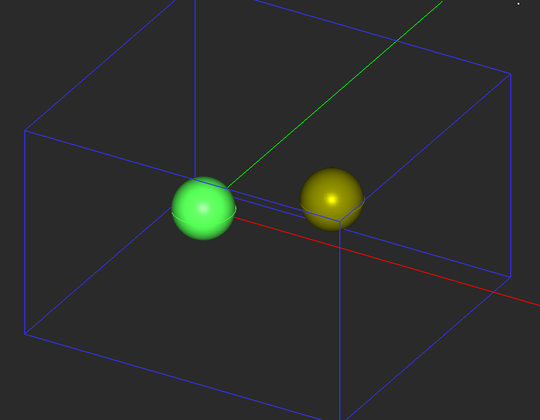The geometry of two dielectric spheres with the same material properties but belonging to two different object groups.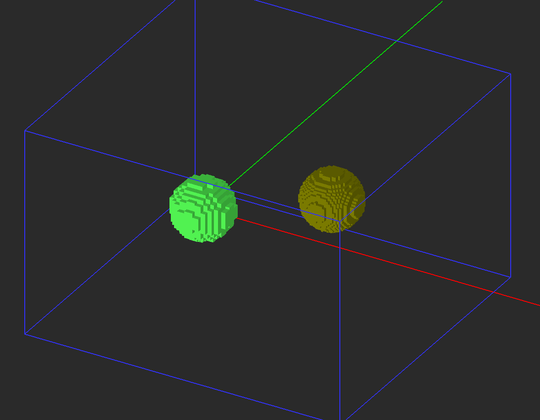The FDTD mesh of the two dielectric spheres. The left sphere is meshed using a global density of 20 cells/λeff, while the right sphere is meshed using a locked density of 100 cells/λeff.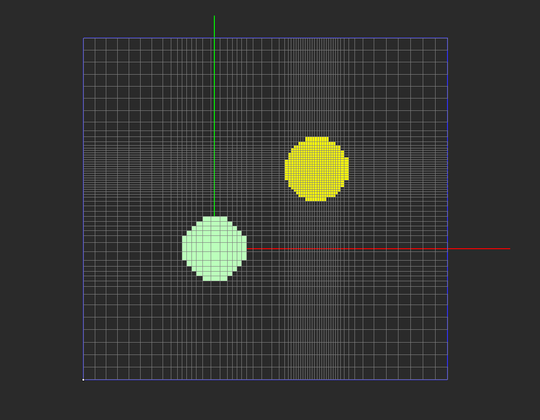The top view of the mesh of the two dielectric spheres also showing the XY grid plane.